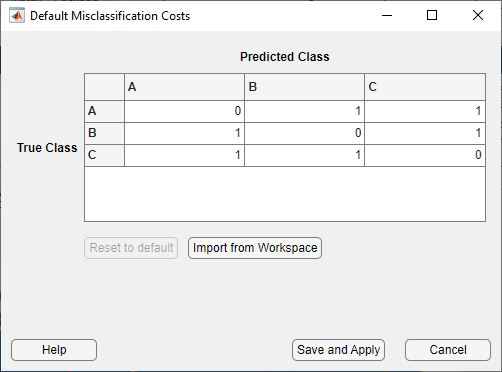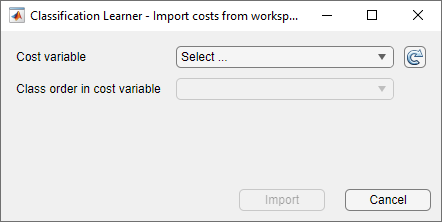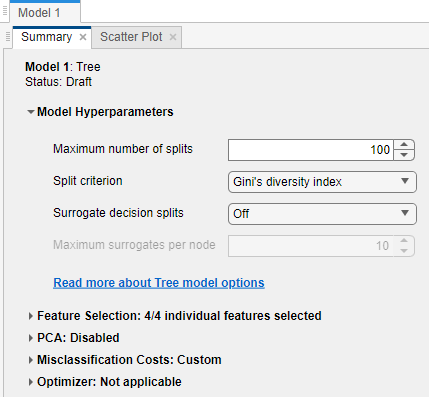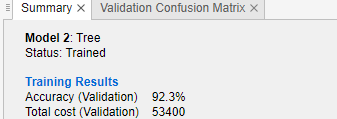## Misclassification Costs in Classification Learner App

By default, the Classification Learner app creates models that assign the same penalty to all misclassifications during training. For a given observation, the app assigns a penalty of 0 if the observation is classified correctly and a penalty of 1 if the observation is classified incorrectly. In some cases, this assignment is inappropriate. For example, suppose you want to classify patients as either healthy or sick. The cost of misclassifying a sick person as healthy might be five times the cost of misclassifying a healthy person as sick. For cases where you know the cost of misclassifying observations of one class into another, and the costs vary across the classes, specify the misclassification costs before training your models.

Note

Custom misclassification costs are not supported for logistic regression models.

### Specify Misclassification Costs

In the Classification Learner app, in the Options section of the Classification Learner tab, select . The app opens a dialog box that shows the default misclassification costs (cost matrix) as a table with row and column labels determined by the classes in the response variable. The rows of the table correspond to the true classes, and the columns correspond to the predicted classes. You can interpret the cost matrix in this way: the entry in row i and column j is the cost of misclassifying ith class observations into the jth class. The diagonal entries of the cost matrix must be 0, and the off-diagonal entries must be nonnegative real numbers.

You can specify your own misclassification costs in two ways: by entering values directly into the table in the dialog box or by importing a workspace variable that contains the cost values.Note

A scaled version of the cost matrix gives the same classification results (for example, confusion matrix and accuracy), but with a different total misclassification cost. That is, if `CostMat` is the misclassification cost matrix and `a` is a positive, real scalar, then a model trained with the cost matrix `a*CostMat` has the same confusion matrix as that model trained with `CostMat`.

#### Enter Costs Directly in Dialog Box

In the misclassification costs dialog box, double-click an entry in the table that you want to edit. Delete the value and type the correct misclassification cost for the entry. When you are done editing the table, click to save your changes. The changes apply to all existing draft models and to any new draft models you create using the Models gallery on the Classification Learner tab.

#### Import Workspace Variable Containing Costs

In the misclassification costs dialog box, click . The app opens a dialog box for importing costs from a variable in the MATLAB® workspace.From the Cost variable list, select the cost matrix or structure that contains the misclassification costs.

• Cost matrix — The matrix must contain the misclassification costs. The diagonal entries must be 0, and the off-diagonal entries must be nonnegative real numbers. By default, the app uses the class order shown in the previous misclassification costs dialog box to interpret the cost matrix values.

To specify the order of the classes in the cost matrix, create a separate workspace variable containing the class names in the correct order. In the import dialog box, select the appropriate variable from the Class order in cost variable list. The workspace variable containing the class names must be a categorical vector, logical vector, numeric vector, string array, or cell array of character vectors. The class names must match (in spelling and capitalization) the class names in the response variable.

• Structure — The structure must contain the fields `ClassificationCosts` and `ClassNames` with these specifications:

• `ClassificationCosts` — Matrix that contains misclassification costs.

• `ClassNames` — Names of the classes. The order of the classes in `ClassNames` determines the order of the rows and columns of `ClassificationCosts`. The variable `ClassNames` must be a categorical vector, logical vector, numeric vector, string array, or cell array of character vectors. The class names must match (in spelling and capitalization) the class names in the response variable.

After specifying the cost variable and the class order in the cost variable, click Import. The app updates the table in the misclassification costs dialog box.

After you specify a cost matrix that differs from the default, the app updates the Summary tab of existing draft models. To open this tab, click in the Models section of the Classification Learner tab. In the Summary pane, the app displays a Misclassification Costs: Custom section. For models that use the default misclassification costs, the app displays a Misclassification Costs: Default section.You can click Misclassification Costs: Custom to expand the section and view the table of misclassification costs.

### Assess Model Performance

After specifying misclassification costs, you can train and tune your models as usual. However, using custom misclassification costs can change how you assess the performance of a model. For example, instead of choosing the model with the best accuracy, choose a model that has good accuracy and a low total misclassification cost. The total misclassification cost for a model is `sum(CostMat.*ConfusionMat,"all")`, where `CostMat` is the misclassification cost matrix and `ConfusionMat` is the confusion matrix for the model. The confusion matrix shows how the model classifies observations in each class. See Check Performance Per Class in the Confusion Matrix.

To inspect the total misclassification cost of a trained model, select the model in the Models pane. On the Classification Learner tab, in the Models section, click . In the Summary tab, look at the Training Results section. The total misclassification cost is listed below the accuracy of the model.### Misclassification Costs in Exported Model and Generated Code

After you train a model with custom misclassification costs and export it from the app, you can find the custom costs inside the exported model. For example, if you export a tree model as a structure named `trainedModel`, you can use the following code to access the cost matrix and the order of the classes in the matrix.

```trainedModel.ClassificationTree.Cost trainedModel.ClassificationTree.ClassNames```

When you generate MATLAB code for a model trained with custom misclassification costs, the generated code includes a cost matrix that is passed to the training function through the `Cost` name-value argument.